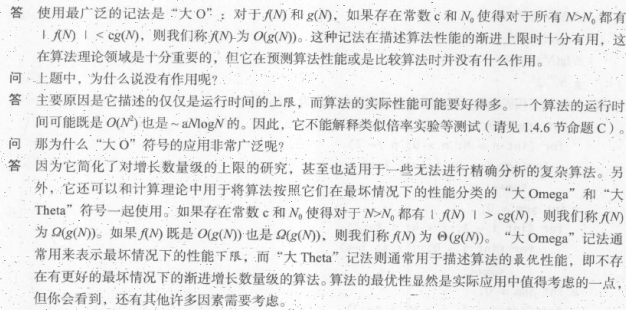# 三、算法分析（three-sum算法）

## 算法分析

### 计时类设计

public class Stopwatch {

private final long start;
public Stopwatch() {
start = System.currentTimeMillis();
}
public double elapsedTime() {
long now = System.currentTimeMillis();
return (now - start) / 1000.0;
}

### 分析

    public static int count(int[] a) {
int N = a.length;
int cnt = 0;
for (int i = 0; i < N; i++) {
for (int j = i+1; j < N; j++) {
for (int k = j+1; k < N; k++) {
if (a[i] + a[j] + a[k] == 0) {
cnt++;
}
}
}
}
return cnt;
} 

### 数学模型

执行每条语句的耗时；



f(N)$~f(N)$表示所有随着N$N$的增大除以f(N)$f(N)$的结果趋近于1的函数。我们用g(N)$g(N)$~f(N)$f(N)$表示g(N)f(N)$g(N)f(N)$随着N$N$的增大趋近于1

g(N)af(N)$g(N) \sim af(N)$
f(N)=Nb(logN)c$f(N)=N^b(logN)^c$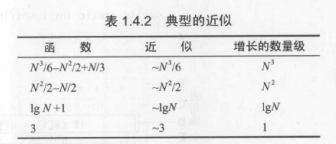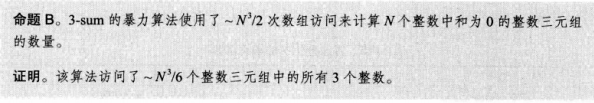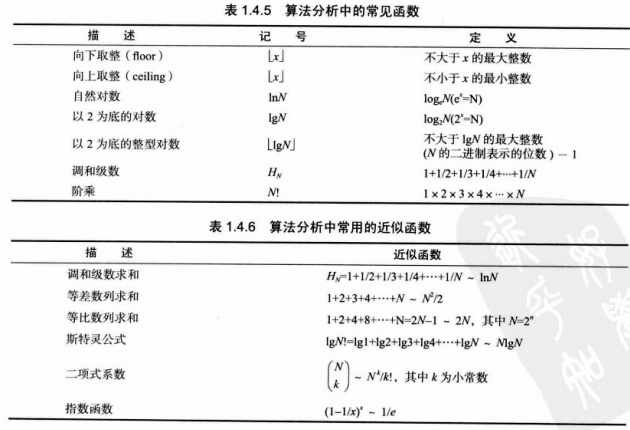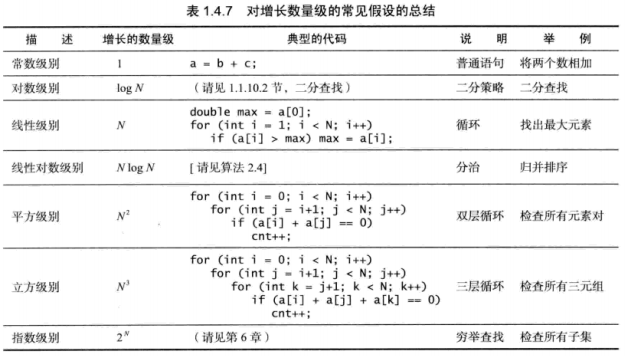### 算法优化

2-sum:先从2-sum推导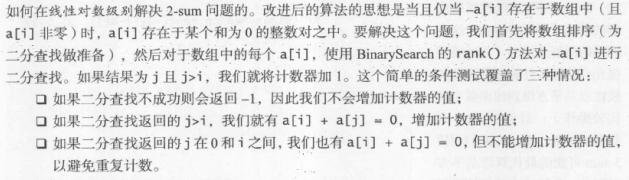public static int count(int[] a){
Arrays.sort(a);
int N = a.length;
int cnt = 0;
for (int i = 0; i < N; i++) {
if(BinarySearch.indexOf(a,-a[i])>1)
cnt++;
}
return cnt;
}

3-sum快速算法

package threesum;
import java.util.Arrays;
import edu.princeton.cs.introcs.In;
import edu.princeton.cs.introcs.StdOut;
public class ThreeSumFast {
// Do not instantiate.
private ThreeSumFast() { }
// returns true if the sorted array a[] contains any duplicated integers
private static boolean containsDuplicates(int[] a) {
for (int i = 1; i < a.length; i++)
if (a[i] == a[i-1]) return true;
return false;
}
public static void printAll(int[] a) {
int N = a.length;
Arrays.sort(a);
if (containsDuplicates(a)) throw new IllegalArgumentException("array contains duplicate integers");
for (int i = 0; i < N; i++) {
for (int j = i+1; j < N; j++) {
int k = Arrays.binarySearch(a, -(a[i] + a[j]));
if (k > j) StdOut.println(a[i] + " " + a[j] + " " + a[k]);
}
}
}
public static int count(int[] a) {
int N = a.length;
Arrays.sort(a);
if (containsDuplicates(a)) throw new IllegalArgumentException("array contains duplicate integers");
int cnt = 0;
for (int i = 0; i < N; i++) {
for (int j = i+1; j < N; j++) {
int k = Arrays.binarySearch(a, -(a[i] + a[j]));
if (k > j) cnt++;
}
}
return cnt;
}
}

### 倍率实验（估计运行时间的方法）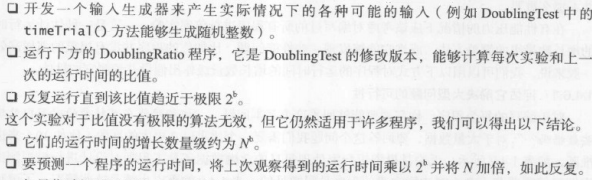three-sum里面的比例为23$2^3$,乘以8就行了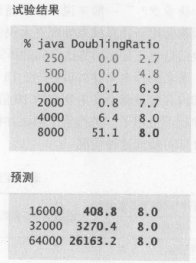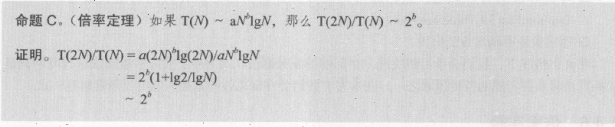（除了指数级别之外都会出现收敛）

1.非首项中的大常熟对结果影响很大
2.非决定性的内循环（即成本模型不是你想象的那样）
3.指令时间（假设时间不对）
4.系统因素
5.对输入的强烈依赖
6.多个问题参量

### 处理对输入的依赖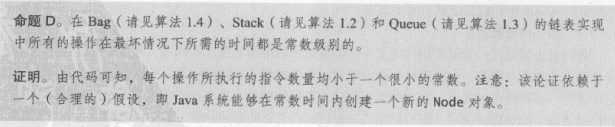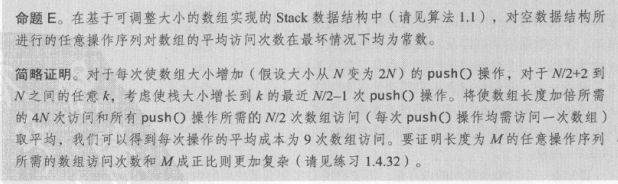### 内存